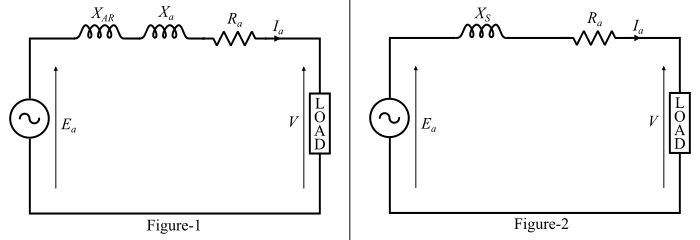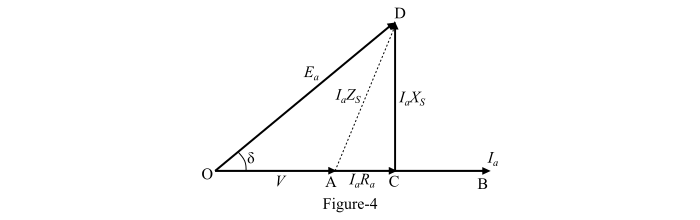# Equivalent Circuit and Phasor Diagram of Synchronous Generator or Alternator

The equivalent circuit of an alternator or synchronous generator is shown in Figure-1. The equivalent circuit of the alternator is redrawn in Figure-2 by taking synchronous reactance $𝑋_{𝑆} = 𝑋_{𝐴𝑅} + 𝑋_{𝑎}$. By using the equivalent circuit, the phasor diagram of the alternator can be drawn as given below.By referring the equivalent circuit, we can write,

$$\mathrm{𝑽 = 𝑬_{𝒂} − 𝑰_{𝒂}(𝑅_{𝒂} + 𝑗𝑋_{𝑆}) … (1)}$$

## Phasor Diagram of Alternator for Lagging Power Factor Load

The phasor diagram of an alternator supplying a load of lagging power factor is shown in Figure-3. Here, the power factor is 𝜑 lagging. To draw this phasor diagram, the terminal voltage V is taken as reference phasor and its direction is along OA where, OA = V.

Since the power factor is 𝜑 lagging, the armature current $𝐼_{𝑎}$ lags behind the voltage V by an angle equal to the power factor angle 𝜑 and the direction of the armature current phasor is along OB, where OB = Ia. The armature resistance $𝑰_{𝑎}𝑅_{𝑎}$ voltage drop is represented by phasor AC and it is in phase with the armature current.

The voltage drop in the synchronous reactance is $𝑰_{𝑎}𝑋_{𝑆}$ is represented by phasor CD and it leads the armature current Ia by 90°, thus the phasor CD is drawn in a direction perpendicular to OB. The total voltage drop in the synchronous impedance is the phasor sum of $𝑰_{𝑎}𝑅_{𝑎}$ and $𝑰_{𝒂}𝑋_{𝑆}$ and it is represented by the phasor AD. The actual generated voltage Ea in the alternator is represented by the phasor OD.The magnitude of the actual generated voltage Ea can be determined from the triangle OFD as follows −

$$\mathrm{𝑂𝐷^{2} = 𝑂𝐹^{2} + 𝐹𝐷^{2} = (𝑂𝐺 + 𝐺𝐹)^{2} + (𝐹𝐶 + 𝐶𝐷)^{2}}$$

$$\mathrm{{𝐸^{2}_{𝑎}} = (𝑉\:cos\:φ + 𝑰_{𝑎}𝑅_{𝑎})^{2} + (𝑉\:sin\:φ + 𝑰_{𝒂}𝑋_{𝑆})^{2}}$$

$$\mathrm{\Rightarrow\:𝐸_{𝑎} = \sqrt{(𝑉\:cos\:φ + 𝑰_{𝑎}𝑅_{𝑎})^{2} + (𝑉\:sin\:φ + 𝑰_{𝒂}𝑋_{𝑆})^{2} … (2)}}$$

## Phasor Diagram of Alternator for Unity Power Factor Load

The phasor diagram of the alternator supplying a load of unity power factor is shown in Figure-4.From the right angled triangle OCD, the magnitude of the actual generated voltage can be determined as follows −

$$\mathrm{𝑂𝐷^{2} = 𝑂𝐶^{2} + 𝐶𝐷^{2} = (𝑂𝐴 + 𝐴𝐶)^{2} + (𝐶𝐷)^{2}}$$

$$\mathrm{{𝐸^{2}_{𝑎}} =(𝑉 + 𝑰_{𝑎}𝑅_{𝑎})^{2} + (𝑰_{𝒂}𝑋_{𝑆})^{2}}$$

$$\mathrm{\Rightarrow\:𝐸_{𝑎} = \sqrt{(𝑉 + 𝑰_{𝑎}𝑅_{𝑎})^{2} + (𝑰_{𝒂}𝑋_{𝑆})^{2}}… (3)}$$

The phasor diagram of the alternator supplying a load of leading power factor is shown in Figure-5.From the right angled triangle OFD, the magnitude of the actual generated voltage can be determined as follows −

$$\mathrm{𝑂𝐷^{2} = 𝑂𝐹^{2} + 𝐹𝐷^{2} = (𝑂𝐺 + 𝐺𝐹)^{2} + (𝐹𝐶 − 𝐶𝐷)^{2}}$$

$$\mathrm{{𝐸^{2}_{𝑎}} =(𝑉\:cos\:φ + 𝑰_{𝑎}𝑅_{𝑎})^{2} + (𝑉\:sin\:φ − (𝑰_{𝒂}𝑋_{𝑆})^{2}}$$

$$\mathrm{\Rightarrow\:𝐸_{𝑎} = \sqrt{(𝑉\:cos\:φ + 𝑰_{𝑎}𝑅_{𝑎})^{2} + (𝑉\:sin\:φ − (𝑰_{𝒂}𝑋_{𝑆})^{2}}… (4)}$$

Here, the angle between the actual generated voltage (Ea) and the terminal voltage (V) is known as power angle or torque angle of the alternator. The power angle (𝛿) changes with the load and is a measure of air-gap power developed in the machine.